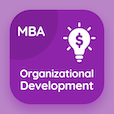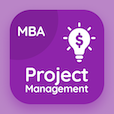MBA Statistics MCQs

MBA Statistics MCQ - Topics

Learn Events and Their Probabilities Multiple Choice Questions (MCQ Quiz), Events and Their Probabilities quiz answers PDF to learn mba statistics course for mba statistics online classes. Introduction to Probability Multiple Choice Questions and Answers (MCQs), Events and Their Probabilities quiz questions for top part time MBA programs. Events and Their Probabilities Book PDF: relationships of probability, events and their probabilities, experiments, counting rules and assigning probabilities test prep for one year online MBA.

"A random variable say x, having some finite range of values is known to be" MCQ PDF: events and their probabilities App APK with continuous random variable, compound random variable, discrete random variable, and transitive random variable choices for top part time MBA programs. Study events and their probabilities quiz questions for merit scholarship test and certificate programs .

## MCQ on Events and Their Probabilities Quiz

MCQ: A random variable say x, having some finite range of values is known to be

Continuous random variable
Compound random variable
Discrete random variable
Transitive random variable

MCQ: The probability that event B will occur, given that event A has occurred, is known to be

Complimentary probability
Compound probability
Conditional probability
Associative probability

MCQ: A coin is tossed 6 times, what is the probability that at least one head will occur?

1 ⁄ 64
6 ⁄ 64
63 ⁄ 64
64 ⁄ 64

MCQ: An event that includes all those events which are not included in A is known to be its

Exclusive event
Complimentary event
Transitive event
Reflective event

MCQ: Assigning numerical values is the output generated from a random experiment, known to be

Expected variable
Random variable
Discrete variable
Continuous variable

### More Quizzes on MBA Statistics Book